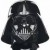# Minitab Multiple Linear Regression Help

Six Sigma – iSixSigma Forums General Forums Tools & Templates Minitab Multiple Linear Regression Help

Viewing 5 posts - 1 through 5 (of 5 total)
• Author
Posts
• #253669shar6580
Participant

I am aiming to assess the effect of BMI (continuous) on certain biomarkers (also continuous) whilst adjusting for several relevant variables (mixed categorical and continuous) using multiple regression. My data is non-normal which I believe violates one of the key assumptions of multiple linear regression. Whilst I think it can still be performed I think it affects significance testing which is an issue for me. I think I can transform the data and then perform regression but I’m not sure and also have some questions regarding the implications of this. I have tried Box-cox transformation but Minitab is unable to do this as some of the values are zero. I have performed Johnson transformation (which I think is a variation of Yeo-Johnson transformation) and now have columns of normal looking transformed data but – A) is this the right thing to do, B) if so do I need to do this for all non-normal variables or just the outcome variable, C) how will this effect the beta coefficient in terms of calculating the final quantitative effect BMI has on the variable given that I’m using transformed data and not the original? I could potentially change my outcome variable to a categorical variety and use multiple logistic regression but not sure if/how this helps. I am not thinking about non-linear regression at this point as this seems more complex (perhaps too much for me) and I’m hoping to solve this issues without in the first instance. Any help would be much appreciated.

0
#253686Ken Feldman
Participant

@shar6580 Double check the correctness of your assumptions. The only assumption for normality is in the residuals. There is no normality assumption for the raw data. Save your efforts of all those transformations.

0
#253687Robert Butler
Participant

Darth is correct. Here’s the relevant quote from a standard statistics text.

Applied Regression Analysis 2nd Edition – Draper and Smith pages 22 and 23

“[with regard to regression] Up to this point we have made no assumptions at all that involve probability distributions. A number of specified algebraic calculations have been made and that is all. We now make the basic assumptions that for a model of Y = fn(Xi +e) (I can’t write epsilon using this platform so I’m using “e” in its place)

1. e is a random variable with mean zero and variance sigma**2.
2. e(i) and e(j) are uncorrelated such that cov(e(i),e(j)) = 0
3. e(i) is a normally distributed random variable, with mean 0 and variance sigma**2″

There are no other assumptions/requirements.

The need for approximate normality in the residuals is because it is the residuals that inform the correctness of the t and F tests used for assessing term significance. In order to address the issue of approximate normality you will need to run a residual analysis – and when you do please follow the guidelines for running a proper analysis (Chapter 3 of the above book has the details) this means assessing the residuals graphically – not just dumping them into some test for normality.

0
#253688Ken Feldman
Participant

@rbutler Robert, as usual, a concise simple to understand explanation LOL. I always learn so much from your posts…at least the parts that I understand. Hope all is well.

0
#253690Robert Butler
Participant

@Darth – things are going well here – how about you and yours?

0
Viewing 5 posts - 1 through 5 (of 5 total)

You must be logged in to reply to this topic.